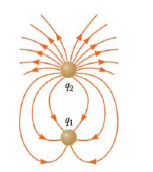Chapter 15, Problem 34P

Chapter
Section
Textbook Problem

Figure P15.31 shows the electric held lines for two point charges separated by a small distance, (a) Determine the ratio q1/q2. (b) What are the signs of q1 and q2?Figure P15.34

(a)

To determine
The ratio q1/q2 .

Explanation

Total number of field lines leaving q2 is 18. Total number of field lines entering q1 is 6. Therefore, the ratio is,

q1q2=6<

(b)

To determine
The signs of the two charges.

Still sussing out bartleby?

Check out a sample textbook solution.

See a sample solution

The Solution to Your Study Problems

Bartleby provides explanations to thousands of textbook problems written by our experts, many with advanced degrees!

Get Started

What is a chemical reaction?

Biology: The Dynamic Science (MindTap Course List)

Define the following terms: a. chromosome b. chromatin

Human Heredity: Principles and Issues (MindTap Course List)

Complete the following reactions: a. b.

Chemistry for Today: General, Organic, and Biochemistry

5.34 Define the term mole fraction

Chemistry for Engineering Students